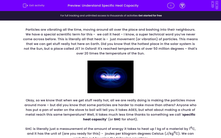# Understand Specific Heat Capacity

In this worksheet, students will explore the idea of heat and calculate the specific heat capacity of a given object.Key stage:  KS 4

GCSE Boards:   AQA Trilogy, AQA, OCR 21st Century, OCR Gateway, Pearson Edexcel, Eduqas, AQA Synergy,

Difficulty level:#### Worksheet Overview

Particles are vibrating all the time, moving around all over the place and bashing into their neighbours. We have a special scientific term for this -  we call it heat - I know, a super technical word you’ve never come across before. This is literally all that heat is -  just movement (or vibration) of particles. This means that we can get stuff really hot here on Earth. Did you know that the hottest place in the solar system is not the Sun, but a place called JET in Oxford! It's reached temperatures of over 50 million degrees – that's over 20 times the temperature of the Sun.Okay, so we know that when we get stuff really hot, all we are really doing is making the particles move around more – but did you know that some particles are harder to make move than others? Anyone who has put a pan of water on the stove to boil will tell you it takes AGES, but what about making a chunk of metal reach this same temperature? Well, it takes much less time thanks to something we call 'specific heat capacity' (or SHC for short).

SHC is literally just a measurement of the amount of energy it takes to heat up 1 kg of a material by 1oC, and it has the unit of (are you ready for this) -  joules per kilogram degrees Celsius (J/kgoC). We can work this out by using the following equation:

energy transferred = mass × specific heat capacity × temperature change

To be really helpful, you will NOT need to remember this equation in the exam – you’ll just need to make sure that you can remember which number is which!

So, let’s go through an example question and how you might answer it:

A block of aluminium has a specific heat capacity of 900 J/kgoC and is heated from a starting temperature of 0 to a final temperature of 100oC. The mass of the block is 4 kg. How much energy is transferred to the object?

You can answer a question like this in stages:

1   Find all of the numbers and highlight them:

A block of aluminium has a specific heat capacity of 900 J/kgoC and is heated from a starting temperature of 0 to a final temperature of 100oC. The mass of the block is 4 kg. How much energy is transferred to the object?

2   Now we need to find out where they all fit into the equation. Write them out and put the corresponding number next to them like this:

Mass = 4 kg

Specific heat capacity = 900 J/kgoC

Temperature change =  From 0 to 100

3   There is a temperature change, so work out what the difference is (super hard right?)

Mass = 4 kg

Specific heat capacity = 900 J/kgoC

Temperature change = 100 – 0 = 100oC

4   Finally, write them into the equation, put the numbers in the calculator and press =

Energy = 4 x 900 x 100

Energy = 360,000 J

Don’t forget your units! And make sure you ALWAYS write out every step – this way the examiner can follow what you are thinking and might be able to give you some marks even if you don’t get the answer right.

### What is EdPlace?

We're your National Curriculum aligned online education content provider helping each child succeed in English, maths and science from year 1 to GCSE. With an EdPlace account you’ll be able to track and measure progress, helping each child achieve their best. We build confidence and attainment by personalising each child’s learning at a level that suits them.

Get started